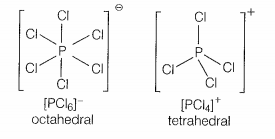In solid-state, PCl5 exists as:
1. Covalent solid.
2. Octahedral structure.
3. Ionic solid with ${\left[{\mathrm{PCl}}_{6}\right]}^{+}$ octahedral and ${\left[{\mathrm{PCl}}_{4}\right]}^{-}$ tetrahedral.
4. Ionic solid with ${\left[{\mathrm{PCl}}_{4}\right]}^{+}$ tetrahedral and ${\left[{\mathrm{PCl}}_{6}\right]}^{-}$ octahedral.

HINT: ${\mathrm{PCl}}_{5}$ generates ions in solid form.
Explanation:
STEP 1: In solid state ${\mathrm{PCl}}_{5}$ exists as an ionic solid with ${\left[{\mathrm{PCl}}_{4}\right]}^{+}$ tetrahedral and ${\left[{\mathrm{PCl}}_{6}\right]}^{-}$ octahedral.In solid state, PCl5 prefer to exist as oppositely charged ions like ${\left[{\mathrm{PCl}}_{4}\right]}^{+}$ and ${\left[{\mathrm{PCl}}_{6}\right]}^{-}$as the ionic bonding enhances the crystalline nature .
STEP 2: The attractive forces in an ionic crystal are much greater than the dipole-dipole or dispersion in crystals of covalent substances.